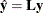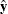# The LOESS Procedure

### Smoothing Matrix

When no iterative reweighting is done, the "Smoothing Matrix" denoted bydefines the linear relationship between the fitted and observed dependent variable values of a loess model. You can obtain the predicted values of a loess fit from the observed values viawhereis the vector of observed values andis the corresponding vector of predicted values of the dependent variable. Note thatis an n by n matrix, where n is the number of observations in the analysis. PROC LOESS does not explicitly formif the DFMETHOD=EXACT option is not explicitly or implicitly selected.